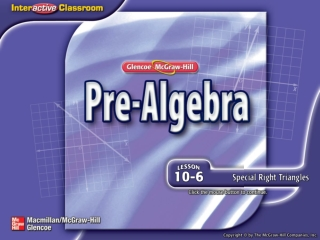DownloadDownload PresentationSplash Screen

# Splash Screen

Download Presentation## Splash Screen

- - - - - - - - - - - - - - - - - - - - - - - - - - - E N D - - - - - - - - - - - - - - - - - - - - - - - - - - -
##### Presentation Transcript

1. Splash Screen

2. Five-Minute Check (over Lesson 10–5) Then/Now Example 1: Find the Hypotenuse in a 45°–45°–90° Triangle Key Concept: 45°–45°–90° Triangles Example 2: Find Missing Measures in a 30°–60°–90° Triangle Key Concept: 30°–60°–90° Triangles Example 3: Real-World Example: Use SpecialRight Triangles Lesson Menu

3. Find the distance between A(2, –3) and B(8, 5). A. 6 B. 8 C. 10 D. 12 5-Minute Check 1

4. Find the distance between C(4, –1) and D(–3, –4). Round to the nearest tenth. A. 6.7 B. 6.8 C. 7 D. 7.6 5-Minute Check 2

5. Find the perimeter of ΔABC.Round to the nearest tenth. A. 23 units B. 24.3 units C. 26.2 units D. 28 units 5-Minute Check 3

6. A distance of 2 units on a map represents 1 block. Caroline’s house is located at (4, –4) and the post office is located at (–3, 6). If Caroline walks diagonally from her house to the post office, how many blocks will she walk? A. 6 B. 6.1 C. 6.2 D. 6.3 5-Minute Check 4

7. A. B. C. D. Which expression can be used to find the distance between K(3, –4) and L(3,–5)? 5-Minute Check 5

8. You used the Pythagorean Theorem to find missing measures in right triangles. (Lesson 10–4) • Find missing measures in 45°-45°-90° triangles. • Find missing measures in 30°-60°-90° triangles. Then/Now

9. Find the Hypotenuse in a 45°-45°-90° Triangle ΔRST is a 45°-45°-90° triangle. Find the length of the hypotenuse. Example 1

10. A. B. C.14 mm D. ΔRST is a 45°-45°-90° triangle. What is the length of the hypotenuse? Example 1

11. Concept A

12. Find Missing Measures in a 30°-60°-90° Triangle ΔPQR is a 30°-60°-90° triangle. Find the exact length of the missing measures. In a 30°-60°-90° triangle, the hypotenuse is 2 times the length of the shorter leg. Example 2

13. A. B. C. D. ΔPQR is a 30°-60°-90° triangle. What are the exact lengths of x and y? Example 2

14. Concept B

15. Use Special Right Triangles GATES A gate has a metal diagonal bar that forms a 30° angle with the bottom edge of the frame. The height of the gate is 2 feet. Find y, the length of the gate. Round to the nearest tenth. Understand You know the length of the shorter leg of a 30°-60°-90° triangle. You need to find the length of the longer leg. Example 3

16. Plan To find y, use the relationship between the shorter leg and the longer leg in a 30°-60°-90° triangle. The longer leg is times the shorter leg. Solve 2nd ENTER × ENTER 3 2 3.464101615 Use Special Right Triangles To find the decimal value of y use a calculator. Example 3

17. Use Special Right Triangles Round to the nearest tenth. Answer: The length of the gate is about 3.5 feet. Check Use the Pythagorean Theorem to check the solution. You know the hypotenuse is 2 ● 2 or 4 feet. Since 22 + 3.52 = 16.25 and 16.25 ≈ 42, the answer is reasonable. Example 3

18. A. B. C.4 ft D. ft ft ft A gate has a metal diagonal bar that forms a 45° angle with the bottom edge of the frame. The height of the gate is 2 feet. What is the length of the diagonal bar? Example 3

19. End of the Lesson